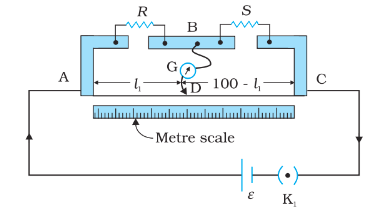# 3.10    (a) In a meter bridge [Fig. 3.27], the balance point is found to be at 39.5 cm from the end A, when the resistor Y is of 12.5 Ω. Determine the resistance of X. Why are the connections between resistors in a Wheatstone or meter bridge made of thick copper strips?Balance Point from the end A, l1 = 39.5 cm

Resistance of Y = 12.5 Ω

We know, for a meter bridge, balance condition is:

The connections between resistors in a Wheatstone or meter bridge made of thick copper strips to minimise the resistance of the connection which is not accounted for in the bridge formula.

Exams
Articles
Questions